Home > PC > Chapter 11 > Lesson 11.2.1 > Problem11-71

11-71.
1. Find the rectangular form of each complex number. Use exact values! Homework Help ✎

1. z = 6 (cos(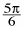) + i sin())

2. z = 7 (cos(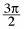) + i sin())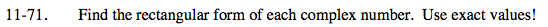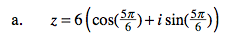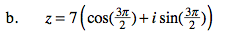Substitute the exact values for sine and cosine of 5π/6.

$\textit{z}=6\left( -\frac{\sqrt{3}}{2}+\frac{1}{2}\textit{i} \right)$

Distribute.

$\textit{z}=-3\sqrt{3}+3\textit{i}$

Recall how you graph complex numbers.Where would this point be in the xy-plane?

$\left( -3\sqrt{3},3 \right)$